# Difference Between Electric Field and Gravitational Field

## Main Difference – Electric Field vs Gravitational Field

In physics, electric and gravitational fields are very important concepts. An electric field is a model which is used to explain influences and behaviors of charges and varying magnetic fields. Electric fields are produced by stationary charge particles and varying magnetic fields. So, neutral particles cannot create electric fields. A gravitational field, on the other hand, is a model which is used to explain gravitational phenomena of masses. Even though neutral particles such as neutrons do not interact via electromagnetic forces, they do via gravitational forces. This is the main difference between electric field and gravitational field. This article tries to describe the difference between electric field and gravitational field in detail.

## What is an Electric Field

In physics, an electric field is a model used to explain or understand the influences and behaviors of charges and varying magnetic fields. In this model, an electric field is represented by field lines. Electric field lines are directed towards negative charges whereas they are directed outwards from positive charges. Electric fields are produced by electric charges or varying magnetic fields. Unlike charges (negative and positive charges) attract each other, like charges (negative- negative or positive- positive) on the other hand, repel.

In the electric field model, several quantities such as electric field intensity, electric flux density, electric potential and Coulomb forces those associated with charges and varying magnetic fields are discussed. The electric field intensity at a given point is defined as the force on a stationary unit test charge particle exerted by electromagnetic forces.

The electric field intensity (E) produced by a point charge particle (Q) is given by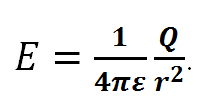where r is the distance between the point and the charged particle and ε is the permittivity of the medium.

Also, the force (F) experienced by a charge q can be expressed as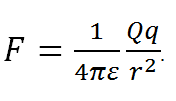r is the distance between two charges

The work done by electromagnetic forces in an electric field is independent of the path. So, electric fields are conservative fields.

The Coulomb’s law can be used to describe an electrostatic field. (An electric field which remains unchanged with time). However, the Maxwell’s equations describe both electric and magnetic fields as a function of charges and currents. So, Maxwell equations are very useful when dealing with electric and magnetic fields.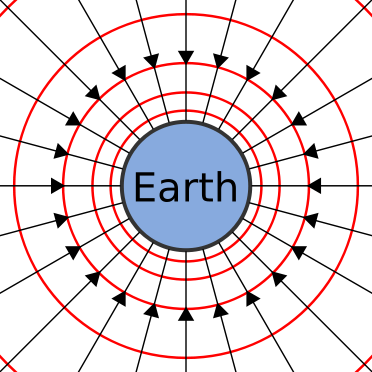Gravitational field lines (black) and equipotentials around the Earth.

## What is a Gravitational Field

The gravitational field is the force field in gravitational interaction which is a model used to explain and understand gravitational phenomena.

In classical mechanics, the gravitational field is a vector field. Several quantities such as gravitational field strength, gravitational force, and gravitational potential are defined in this model. The gravitational field strength at a given point is defined as the force on unit test mass exerted by the gravitational force. The gravitational field strength (g) caused by a mass M at a given point is a function of the position of the point. It can be expressed as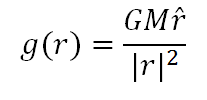G is the universal gravitational constant and rˆ is the unit vector in the direction of r. The mutual gravitational force between two masses M and m is given by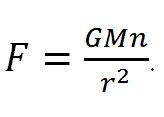Gravitational fields are also conservative force fields since the work done by gravitational forces is independent of the path.

Newtonian theory of gravitation is not a very accurate model. Especially, Newtonian solutions deviate notably from the actual values when dealing with high gravity problems. So, Newtonian theory of gravitation is useful only when dealing with low gravity problems. However, it is accurate enough to be used in most of the practical applications. When dealing with high gravity problems, general relativity must be used. In low gravity, it is approximated to Newtonian theory.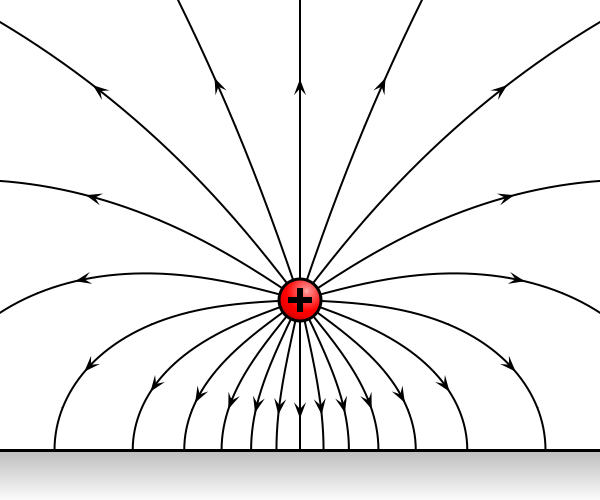Field of a positive electric charge in front of a horizontal perfectly conducting metal surface.

## Difference Between Electric Field and Gravitational Field

### Fields are caused by:

Electric field: Electric field is caused by charges or varying magnetic fields.

Gravitational field: Gravitational field is caused by masses.

### Feld strength in a radial field:

Electric field: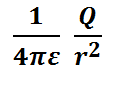Gravitational field: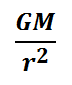### SI unit of the field strength:

Electric field: Vm-1 (NC-1)

Gravitational field: ms-2 (Nkg-1)

### Proportionality Constant:

Electric field: 1/4πε (Depends on the medium as  depends on the medium)

Gravitational field: G (Universal gravitational constant)

### Nature of the force:

Electric field: Either attractive or repulsive. (Arises between charged particles)

Gravitational field: Always attractive. (Arises between masses)

### Force in a radial field:

Electric field: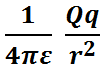(Coulomb’s law)

Gravitational field: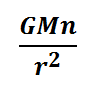(Newton’s law)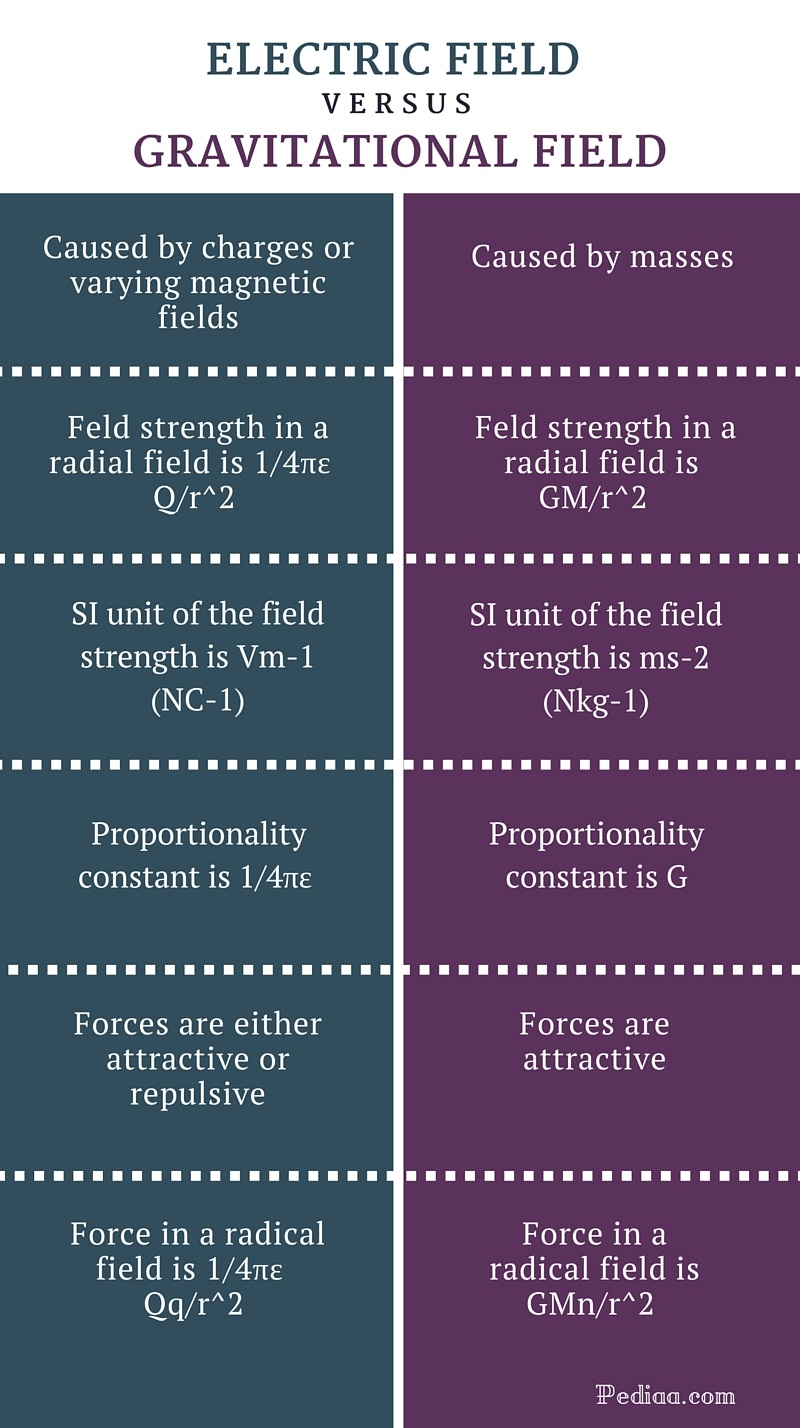Image Courtesy:

“Electric Field” by Geek3 – Own work This plot was created with Vector Field Plot, (CC BY-SA 3.0) via Commons Wikimedia

“Gravitational Field” by Sjlegg – Own work, (Public Domain) via Commons Wikimedia